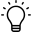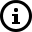# Calibrating a Model 615 Indicator using a Custom SensitivityThis is the best method to calibrate a 615 if using any loads cells with a known output.

1.      Press the hidden programming key for 5 seconds until "615" is displayed.Some Model 615's may have NET/GROSS buttons that replace the hidden arrow keys.

2.      Press SETUP to display "Conf".
3.      Press PRINT/SELECT to display the current config number.
4.      The new configuration number will be 19_00.  Fill in the third digit with the corresponding column number from the table below based on the desired increments.5. Enter the new configuration number by using the hidden up arrow to increase the selected digit and the hidden left arrow to move to the next digit.
7. Press SETUP to display "CuSt".
8. Press PRINT/SELECT display "UPPr"
9. Press PRINT/SELECT to view the first four digits of the current sensitivity.
10. Determine new sensitivity value.  The sensitivity value is equal to the eight digit mV/V output of all the load cells at the capacity used in step 4.
The 8 digit format is n.nnnnnnn with the first digit not shown if it is a zero. If the sensitivity is 0.1234567 then UPPR is 123 and Lo is 4567.
11. Use the same entry method as in step 5 to enter the first four digits of the sensitivity.
12. Press PRINT/SELECT display "UPPr"
13. Press SETUP to display "Lo".
14. Press PRINT/SELECT to view the last four digits of the current sensitivity.
15. Use the same entry method as earlier to enter the last four digits of the sensitivity.
16. Press PRINT/SELECT to display "Lo".
17. Press SETUP to display "CAP".
18. Press PRINT/SELECT to view the current capacity row.
19. Press the hidden up arrow key to enter the row number corresponding to the capacity of the system.20. Press PRINT/SELECT, then SETUP and then ZERO to return to weighing mode.The sensitivity value can be fine tuned using a known weight.  Use the formula: (Observed Weight/Known Weight) x Current Sensitivity = New Sensitivity Value
Example:
The known Weight is 1200 lbs but the scale reads 1000 lbs.
The current UPPr is set to 349 and the Lo is set to 4933.  This means the sensitivity is currently set to 0.3494933.

(1000/1200) x 0.3494933 = 0.2912444

New UPPr = 291      New Lo = 2444